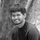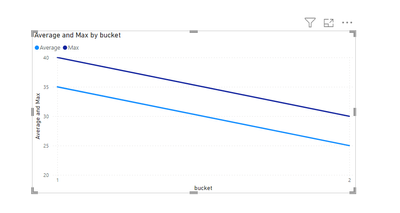cancel
Showing results for
Did you mean:New Member

## average and max of grouped sum by two columns

I have a dataset with three columns as follows. i want to first grouped data by two columns day and bucket and sum over values and second calculate average and max of sums over buckets. I would appreciate if you can help me to figure this out by adding a measure to my table to calculate this.

 day bucket value 1/1/2023 1 10 1/1/2023 1 20 1/1/2023 2 5 1/1/2023 2 15 1/2/2023 1 30 1/2/2023 1 10 1/2/2023 2 25 1/2/2023 2 5 day bucket value 1/1/2023 1 30 1/1/2023 2 20 1/2/2023 1 40 1/2/2023 2 30 bucket value 1 35 2 25 bucket value 1 40 2 30
1 ACCEPTED SOLUTIONSuper User

Can you try this measures?

1. Average

``````Average =
VAR _temp = SUMMARIZE(
Test_max_avg,
Test_max_avg[day],
Test_max_avg[bucket],
"@Value",SUM(Test_max_avg[value]))
VAR _result = AVERAGEX(_temp,[@Value])
RETURN _result``````

2. Max

``````Max =
VAR _temp = SUMMARIZE(
Test_max_avg,
Test_max_avg[day],
Test_max_avg[bucket],
"@Value",SUM(Test_max_avg[value]))
VAR _result = MAXX(_temp,[@Value])
RETURN _result``````

Result:Thanks,

Arul

Regards,
Arul
If I answered your question kindly mark my post as a solution and a kudo would be appreciated.
4 REPLIES 4New Member

Thank you so much Arul, it works.New Member

I want to use bucket in x axis and average and max in y axis of a line chart.Super User

Can you try this measures?

1. Average

``````Average =
VAR _temp = SUMMARIZE(
Test_max_avg,
Test_max_avg[day],
Test_max_avg[bucket],
"@Value",SUM(Test_max_avg[value]))
VAR _result = AVERAGEX(_temp,[@Value])
RETURN _result``````

2. Max

``````Max =
VAR _temp = SUMMARIZE(
Test_max_avg,
Test_max_avg[day],
Test_max_avg[bucket],
"@Value",SUM(Test_max_avg[value]))
VAR _result = MAXX(_temp,[@Value])
RETURN _result``````

Result:Thanks,

Arul

Regards,
Arul
If I answered your question kindly mark my post as a solution and a kudo would be appreciated.Super User

In which visualization you want to show this and what are the columns that you are going to use in the visualization?

Thanks,

Arul

Regards,
Arul
If I answered your question kindly mark my post as a solution and a kudo would be appreciated.